# Equations of Circle

In this article, you will learn about distance formula , midpoint , and equations of circle. The circle is a geometric shape that has a special significance in mathematics. To study the circle, in algebraic form, we need to define it in terms of coordinates in a 2d coordinate system also known as Cartesian coordinate system.

### Coordinate System

You are familiar with the 2d coordinate system already from previous articles. The 2d coordinate system define an point in terms of an ordered pair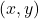from origin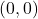. The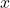represents horizontal distance from 0 and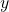represents vertical distance from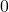.

In the figure 1 above, the point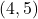is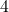units in horizontal direction fromand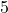units in vertical direction from. Therefore, we must study the circle as a set of points in this 2d coordinate system.

### Distance Formula

The distance formula is derived from Pythagorean theorem to find the distance between two points in the coordinate system. Suppose that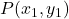and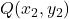are two points in the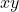plane.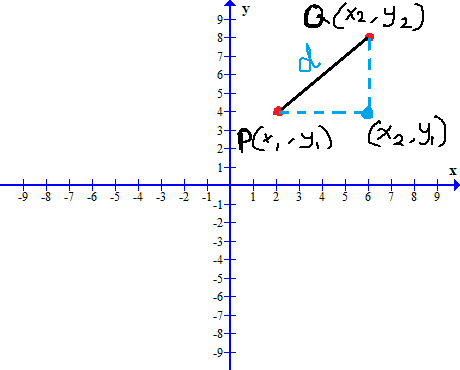Figure 2 – The distance formula can be applied for two points P and Q because to reach point Q from P, we make a triangular shape.

The distance between pointto pointinvolve change ofvalue from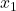to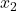value and change invalue from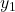to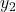. This forms a right triangle in theplane.

The distance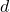is hypotenuse of this right triangle and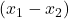and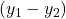are the lengths of sides of this right triangle. Therefore, by Pythagorean theorem,

\begin{aligned}
&d^2 = (x_1 - x_2)^2 + (y_1 - y_2)^2\\ \\
&Take \hspace{2mm} square \hspace{2mm} root \hspace{2mm} of \hspace{2mm} both\hspace{2mm}  sides. \\ \\
&\sqrt{d^2}= \sqrt{(x_1 - x_2)^2 + (y_1 - y_2)^2} \\ \\
&d =\sqrt{(x_1 - x_2)^2 + (y_1 - y_2)^2}
\end{aligned}

The distance formula is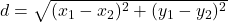.

Note that the lengthor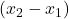does not matter as long as we take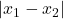or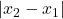. Similarly, we must take positivevalue, which isor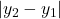.

Example #1

Find the distance between point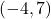and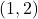.

Solution:

Solution:

\begin{aligned}
&Let \hspace{1mm} x_1 = -4 \hspace{1mm} and \hspace{1mm} y_1 = 7,\hspace{1mm}\\
&also \hspace{1mm}x_2 = 1 \hspace{1mm}and\hspace{1mm} y_2 = 2. \hspace{1mm}Then, \\
&(-4) - 1| = |-5| = 5 \hspace{1mm} and \hspace{1mm} |7-2| = |5| = 5
\end{aligned}

The distance betweenandis:

\begin{aligned}
&d = \sqrt{5^2 + 5^2}\\ \\
&d = \sqrt{50}\\ \\
&d = \sqrt{25 \cdot 2}\\ \\
&d = \sqrt{25} \cdot \sqrt{2}  \hspace{1cm} By Rule \sqrt{ab} = \sqrt{a} \cdot \sqrt{b}, {a,b} > 0\\ \\
&d = 5\sqrt{2}
\end{aligned}

### Mid-Point Formula

Given any two point on theplane, you can find the mid-point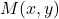. Ifand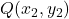are two points on theplane.

Then the mid-point formula is given as

\begin{aligned}
M(x, y) = \left( \frac{x_1 + x_2}{2},\frac{y_1 + y_2}{2}\right )
\end{aligned}

Example #2

Find the mid-pointof points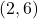and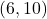.

Solution:

We can easily find the mid-pointusing the mid-point formula, which is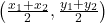. Given points –and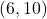.

\begin{aligned}
&Let \hspace{2mm} x_1 = 2, \hspace{2mm}  and \hspace{2mm}  x_2 = 6\\ \\
&Similarly, \\ \\
&y_1 = 6,\hspace{2mm}  and \hspace{2mm} y_2 = 10\\ \\
&Using \hspace{2mm} mid-point \hspace{2mm} formula, \hspace{2mm}  get \\ \\
&M(x, y) = \frac{2 + 6}{2}, \frac{6 + 10}{2}\\ \\
&=\frac{8}{2},\frac{16}{2}\\ \\
&=(4,8)
\end{aligned}

Therefore, the mid-point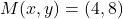.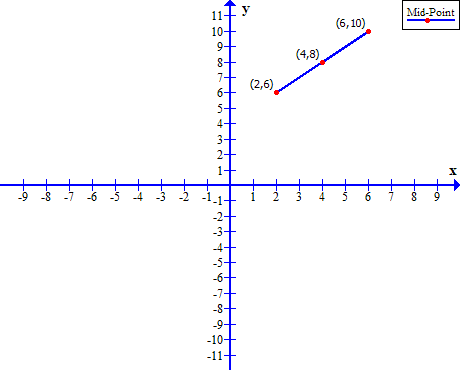Figure 4 – Mid point of (2, 6) and (6, 10) is the point (4, 8).

### Standard Equation of Circle

A circle on theplane is set of all points that equidistant from a fixed point called the center of the circle. The equal distance between center and any point on the circle is called a radius.

Consider the circle in figure 5, with radius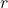and center at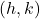. The pointlies on the circle. Therefore, by distance formula, we know that,

\begin{aligned}
&r = \sqrt{(x-h)^2 + (y - k)^2}\\
\end{aligned}

This is the standard equation of a circle with center at.

Circle With Center At The Origin

In the co-ordinate system, the origin is point. A circle whose center is at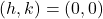has the following equation.

\begin{aligned}
&r = \sqrt{(x - 0)^2 + (y - 0)^2}\\ \\
&r = \sqrt{x^2 + y^2}\\ \\
&or\\ \\
&r^2 = x^2 + y^2
\end{aligned}

Example #3

Find the radius of a circle whose center is atand the point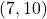lies on the circle.

Solution:

Given center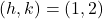and a point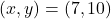, we can use the standard equation of the circle to find the length of radius.

\begin{aligned}
&r = \sqrt{(x-h)^2 + (y - k)^2}\\ \\
&r = \sqrt{(7-1)^2 + (10-2)^2} \\ \\
&r = \sqrt{6^2 + 8^2}\\ \\
&r = \sqrt{36 + 64}\\ \\
&r = \sqrt{100} \\ \\
&r = 10 \hspace{2mm}
\end{aligned}

Therefore, the length of radius is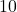for circle whose center is atandis a point on the circle.

Example #4

Find the centerof the circle whose radiusand the point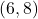. Also,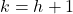.

Solution:

We are given the value of radius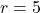and the point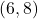on the circle. To find the center pointof the circle, we must use the distance formula which is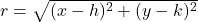and the value of\begin{aligned}
&r = \sqrt{(x-h)^2 + (y-k)^2}\\ \\
&5= \sqrt{(6 - h)^2 + (8-k)^2}\\ \\
&But, \hspace{2mm} k = h + 1 \\ \\
&5= \sqrt{(6 - h)^2 + (8-(h+1))^2}\\ \\
&5 = \sqrt{(6 - h)^2+ (7 - h)^2)}\\ \\
&5 = \sqrt{36 - 12h + h^2 + 49 - 14h + h^2}\\ \\
&5 = \sqrt{85 - 26h + 2h^2 }\\ \\
&Square \hspace{2mm} both\hspace{2mm}  sides \\ \\
&25 = 85 - 26h + 2h^2 \\ \\
&2h^2 - 26h + 85 - 25 = 0\\ \\
&2h^2 - 26h + 60 = 0\\ \\
&h^2 - 13h + 30 = 0 \\ \\
&(h - 3) (h - 10) = 0\\ \\
&By \hspace{2mm} zero\hspace{2mm}  product \hspace{2mm} property\\ \\
&\therefore x = 3 \hspace{2mm} or \hspace{2mm}  x = 10
\end{aligned}

We try to use the value ofin the equation. It seems that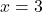seems more appropriate. Let us verify.

\begin{aligned}
&5 = \sqrt{(6-3)^2+(8-4)^2}\\ \\
&5 = \sqrt{3^2 +4^2 }  \hspace{1cm}  \because k = h + 1 = 3 + 1 = 4\\ \\
&5 = \sqrt{9 + 16}\\ \\
&5 = \sqrt{25 }\\ \\
&\therefore 5 = 5
\end{aligned}

### General Equation Of Circle

Suppose a circle has center at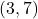with a point on the circle. Therefore, we can find the standard equation of the circle using,

\begin{aligned}
&r^2 = (x - h)^2 + (y - k)^2\\ \\
&r^2 = (x - 3)^2 + (y - 7)^2\\ \\
&r^2 = x^2 - 6x + 9 + y^2 - 14y + 49\\ \\
&r^2 = x^2 + y^2 - 6x - 14y + 58
\end{aligned}

Therefore, the general equation of circle is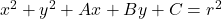where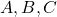are real constants.

Example #5

Given the general equation of circle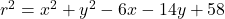find the coordinates for centerof the circle.

Solution:

Given general equation of circle. We will solve it step-by-step. First group the similar variables together and move constant to the right side of the equation.

\begin{aligned}x^2 - 6x + y^2 -14y = - 58\end{aligned}

Now solve forandby completing the square. Note that you have to add same value on both side of the equation.

[\begin{aligned}(x^2 - 6x + 9 ) + (y^2 - 14y + 49) = - 58 + 9 + 49\end{aligned}

The equation is in standard form and we can find the value ofeasily.

[\begin{aligned}(x - 3)^2 + (y - 7)^2 = 0^2\end{aligned}

The center of the circle is at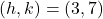.